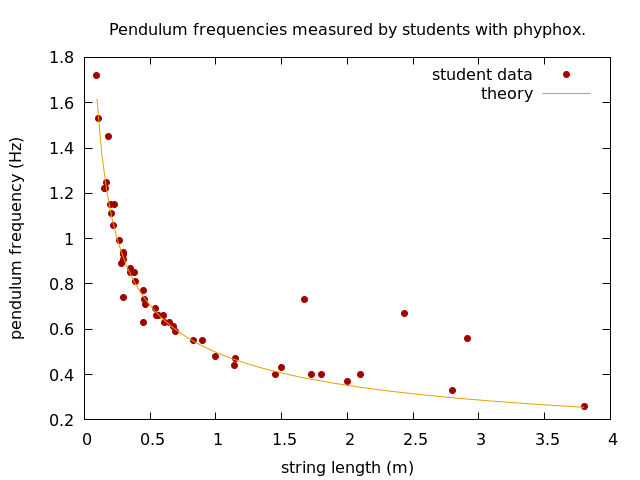# Collective measurement of pendulum frequencies

As part of an optional assignment, we asked our students to create a pendulum, measure its frequency with phyphox and submit the results via a web form. I just picked up the data and got thrilled as the result is amazing:I just had to clean out some obvious cases in which students did not use the correct units (no, they did not build a 69m pendulum).

They did not even know anything about the math of oscillations. Instead, I will introduce this topic in tomorrow’s lecture and will then use their own data to verify the result for the frequency of a pendulum:
$latex f = \frac{\omega}{2\pi} = \frac{1}{2\pi}\sqrt{\frac{g}{l}}&bg=404040&fg=ffffff&s=3$

Contact: contact@phyphox.org
Phyphox has been created at the 2nd Institute of Physics of the RWTH Aachen University.